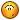BrainDen.com - Brain Teasers

## Question

Put four different whole numbers from 1 to 49, in the corners, then subtract the smaller from the larger and put the answers in between. Keep doing this until all four numbers become equal. How many steps can you make it last? The demo is "(14, 30, 18, 37) lasts 3 steps (don't count the step where they produce the same number)." Find the longest lasting case.

DEMO:

stage 0

14 30 ----> 30-14

37-14 30-18

37 18 37-18

stage 1

16 16-13 16-12

13 12

19 19-13 19-12

stage 2

3 4

6 7

stage 3

1

3 3

1

Stage 4 is stasis so it doesn't count

## Recommended Posts

• 0

I cheated; with less than 6 million different starting combinations, I wrote some code to brute force the answer.

1

8 45

21

Lasted for 12 iterations (possibly 11 or 13, counting isn't my strong suit)

##### Share on other sites

• 0

Is the example two steps?

14 30 16 7 4
=> 23 12 =>
37 18 19 4 7

Are they all just two steps?

a b |a-b| |b-c| |a-d|

=> |c-a| |b-d| =>

c d |d-c| |a-d| |b-c|

##### Share on other sites

• 0

I cheated; with less than 6 million different starting combinations, I wrote some code to brute force the answer.

1

8 45

21

Lasted for 12 iterations (possibly 11 or 13, counting isn't my strong suit)

13 DIAGONAL 1 8 21 45

1 8 21 45

7 13 24 44 - 1

6 11 20 37 - 2

5 9 17 31 - 3

4 8 14 26 - 4

4 6 12 22 - 5

2 6 10 18 - 6

4 4 8 16 - 7

0 4 8 12 - 8

4 4 4 12 - 9

0 0 8 8 - 10

0 8 0 8 - 11

8 8 8 8 - 12

0 0 0 0 - 13

##### Share on other sites

• 0

I cheated; with less than 6 million different starting combinations, I wrote some code to brute force the answer.

1

8 45

21

Lasted for 12 iterations (possibly 11 or 13, counting isn't my strong suit)

Can you share the code?

##### Share on other sites

• 0

Can you share the code?

I'm sure there are more elegant ways of coding this, and it only gives back the first longest set it finds (it won't return 8,21,45,1 after it finds 1,8,21,45) so I'm sure there is more than one answer.

I think 5,29,42,49 might be another set, but haven't tested them yet.

```int a,b,c,d,a1,b1,c1,d1,a2,b2,c2,d2,a3,b3,c3,d3;
int z3=0,z,x;

int main()
{
for(a=1; a<50; a++)
{
for(b=1; b<50; b++)
{
for(c=1; c<50; c++)
{
for(d=1; d<50; d++)
{
a1=a;b1=b;c1=c;d1=d;
x=1;z=0;

while(x==1)
{
if(a1==b1 and c1==d1 and a1==c1)
{
x=0;
}else
{
a2=sqrt ((a1-b1)*(a1-b1));
b2=sqrt ((c1-b1)*(c1-b1));
c2=sqrt ((c1-d1)*(c1-d1));
d2=sqrt ((a1-d1)*(a1-d1));
z+=1;
}

if(a2==b2 and c2==d2 and a2==c2)
{
x=0;
}else
{
a1=sqrt ((a2-b2)*(a2-b2));
b1=sqrt ((c2-b2)*(c2-b2));
c1=sqrt ((c2-d2)*(c2-d2));
d1=sqrt ((a2-d2)*(a2-d2));
z+=1;
}

}
if(z>z3)
{
z3=z;
a3=a;
b3=b;
c3=c;
d3=d;
}

}
}
}
}

cout<<z3<<endl<<endl<<"  "<<a3<<endl<<b3<<"  "<<d3<<endl<<"  "<<c3<<endl;
return 0;
}

```

##### Share on other sites

• 0

Just realized the section:

a1=sqrt ((a2-b2)*(a2-b2));

b1=sqrt ((c2-b2)*(c2-b2));

c1=sqrt ((c2-d2)*(c2-d2));

d1=sqrt ((a2-d2)*(a2-d2));

should actually be:

a1=sqrt ((a2-d2)*(a2-d2));

b1=sqrt ((a2-b2)*(a2-b2));

c1=sqrt ((c2-b2)*(c2-b2));

d1=sqrt ((c2-d2)*(c2-d2));

I don't think it really it effects the outcome, since it stayed in the same order. But still.

##### Share on other sites

• 0

Is the example two steps?

14 30 16 7 4

=> 23 12 =>

37 18 19 4 7

Are they all just two steps?

a b |a-b| |b-c| |a-d|

=> |c-a| |b-d| =>

c d |d-c| |a-d| |b-c|

yes, your example is 2 steps.

##### Share on other sites

• 0

Is the example two steps?

14 30 16 7 4

=> 23 12 =>

37 18 19 4 7

Are they all just two steps?

a b |a-b| |b-c| |a-d|

=> |c-a| |b-d| =>

c d |d-c| |a-d| |b-c|

my example though shows 3 steps

##### Share on other sites

• 0

I cheated; with less than 6 million different starting combinations, I wrote some code to brute force the answer.

1

8 45

21

Lasted for 12 iterations (possibly 11 or 13, counting isn't my strong suit)

##### Share on other sites

• 0

Is the example two steps?

14 30 16 7 4

=> 23 12 =>

37 18 19 4 7

Are they all just two steps?

a b |a-b| |b-c| |a-d|

=> |c-a| |b-d| =>

c d |d-c| |a-d| |b-c|

yes, your example is 2 steps.

It's not my example.

Check 37-14.

##### Share on other sites

• 0

Is the example two steps?

14 30 16 7 4

=> 23 12 =>

37 18 19 4 7

Are they all just two steps?

a b |a-b| |b-c| |a-d|

=> |c-a| |b-d| =>

c d |d-c| |a-d| |b-c|

yes, your example is 2 steps.

It's not my example.

Check 37-14.

silly me.## Join the conversation

You can post now and register later. If you have an account, sign in now to post with your account.×   Pasted as rich text.   Paste as plain text instead

Only 75 emoji are allowed.

×   Your previous content has been restored.   Clear editor

×   You cannot paste images directly. Upload or insert images from URL.